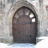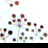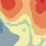# Line barriers: need to increase, NOT scale cost

568
2
09-13-2013 03:46 PMNew Contributor
I know newer versions of Arc allow for scaled cost line barriers.  However, I don't want to scale the cost, I simply want to increase it with a constant value.  Is that possible or is there a particular workaround?
Tags (2)
2 RepliesbyEsri Contributor
Hello, Sherwin.

Point barriers that prohibit travel on edges have existed for a long time. But you're right, at ArcGIS 10.0, line and polygon barriers were added to the mix. Furthermore, point barriers were enhanced to add cost, rather than completely block travel, along an edge. Similarly, line and polygon barriers could either block travel or scale it. Note that point barriers can increase cost by addition and point and line barriers increase by multiplication.

So if you want to add cost every time a barrier is crossed, use added-cost point barriers. I figure you're looking at line barriers because you already have line data, so what you'd need to do is find where your lines cross roads, then turn those crossings into points. The Intersect GP tool is one option for that. (Make sure you set the Output Type parameter on Intersect to points). Once you have your points, you can assign added cost values to the points and load them into your network analysis layer.

If you already have point data, you wouldn't need to run Intersect of course. You could load the points directly.

Best,
RobertbyEsri Contributor
Hello, Sherwin!

When you think about it, the scale barriers, since they intersect a line in two dimensions can't really add a cost.  Robert's point barrier suggestion is the best choice, probably (depending on what your use-case is).

Why are you interested in making additive line barriers, out of curiosity?  I'd love to hear your use-case.

If you know exactly what the cost along an edge is, you could kind-of make it additive.  Your barrier scale factor would equal the quantity of the cost of your edge plus your new cost, divided by the cost of your edge.

Like this:

Scale factor = (additive cost + original edge cost) / original edge cost

That could get complicated, though, depending on how the costs for your edge are determined.  Also, if you only traverse part of an edge, you will get incorrect apportionment.  For example, if you travel 0.5 times the length of the edge, you will still get the full additive cost included.